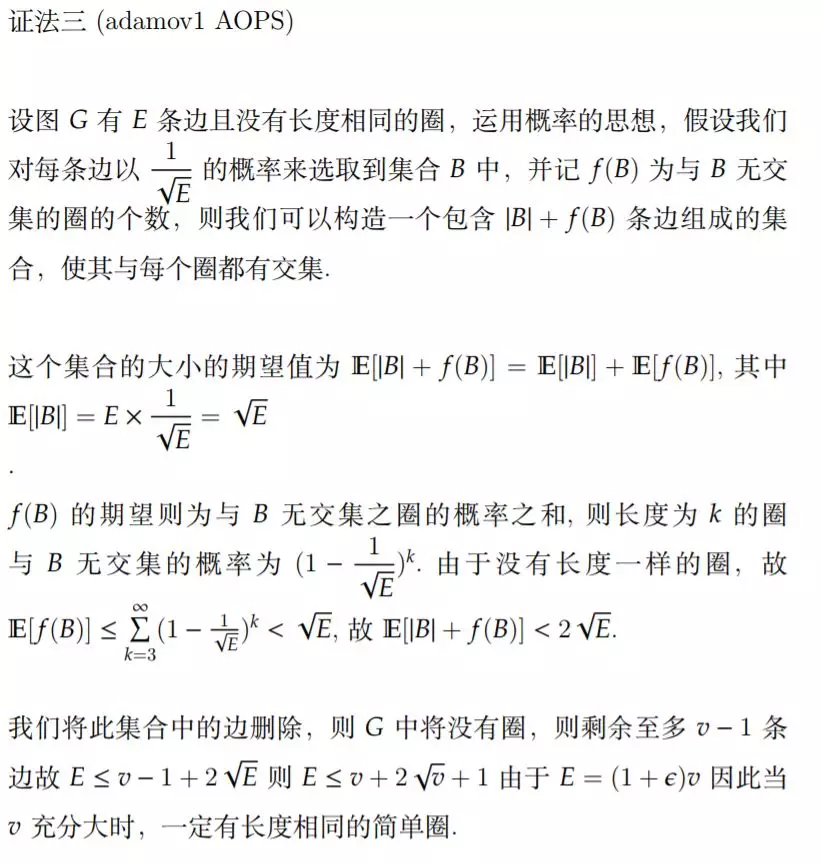# 2019 年罗马尼亚数学大师赛第三题

### 2、大师赛第三题

#### 2.1、第三题原题

Problem 3. Given any positive real number $\epsilon$, prove that, for all but finitely many positive integers $v$, any graph on $v$ vertices with at least $(1 + \epsilon)v$ edges has two distinct simple cycles of equal lengths.

(Recall that the notion of a simple cycle does not allow repetition of vertices in a cycle.)

Russia, Fedor Petrov

#### 2.2、第三题的官方解答

https://mp.weixin.qq.com/s/nZbym4t9rOKFP-5ROluL7g这里给出了翻译，其中的概率解法尤其精妙。### 3、题目背景

RMM problem3 就是这个问题的弱化版本，证明上界小于$n+\epsilon n$。这是俄罗斯出的题目。

Q. E. D.